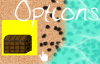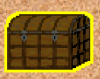#### TotallyAverageGamez

##### Member
Hey All,

Have a question about shader code. I am trying to create an outline shader that draws a border (highlight) 1px wide around an object. The code I am using so far is this:

varying vec2 v_vTexcoord;
varying vec4 v_vColour;

uniform vec4 outlineColor;

void main()
{
vec4 originalColor = v_vColour * texture2D( gm_BaseTexture, v_vTexcoord);
vec4 newColor = outlineColor;
newColor.a = 1.0;
if(originalColor.a != 0.0)
{
newColor = originalColor;
}
else if(texture2D(gm_BaseTexture, v_vTexcoord + vec2(1.0, 0.0)).a != 0.0)
{
newColor = outlineColor;
}
gl_FragColor = newColor;
}​

The logic goes:
-If there is a color already here (alpha != 0.0) leave the pixel alone.
-else if the pixel one to the right (x +1) has an alpha, change to the outline color (yellow).
I plan on doing -x, +y, and -y once I get +x working. The thing is for some reason the line:

if(texture2D(gm_BaseTexture, v_vTexcoord + vec2(1.0, 0.0)).a != 0.0)
Is always returning true and the entire sprite (outside of the original pixels) is completely highlighted yellow.I have the sprite on its own texture page with plenty of border and the automatic crop option disabled in the texture group settings as well.

Am I just not using the texture2D function correctly? Or is there some other way I should be doing this? Any and all help appreciated!

Thanks!

J

#### jacobandgeckos

##### Guest
had a similar problem when I was making a cloud pixel shader.
I remember being thoroughly confused as to why what I was doing wasn't working.
It was resolved in this thread:

Here's what I was missing:
The shader does not automatically know how big a pixel is.
You have to pass in how wide and how tall a pixel is as a both as fractions of one (one being the whole width or height of the shader.)

My shader was 50 pixels wide and 30 pixels tall, so I used this code:
Draw Event:
Code:
``````var outlineW = shader_get_uniform(shdr_cloudepth,"outlineW");
(pass width and height as fraction of 1 into the shader)

Code:
``````vec2 offset;
offset.xy = vec2(outlineW, outlineH);``````
(this sets how big a pixel is in the shader)

and (later in the Pixel Shader):
Code:
``if(texture2D(gm_BaseTexture, v_vTexcoord+3.0*offset).a==0.0)``
(the 3*offset checks for the transparency of a pixel 3 pixels down to the right of the current pixel.)

offset.x and offset.y are the width and the height of the pixel in terms that the shader can understand (1/50 and 1/30)

The full code is in the other thread if you want to see it. It is not exactly for an outline but it does detect transparent pixels. I hope this helps, but I'm not really a shader expert - so if it doesn't, I may be as lost as you.
Good luck!

•TotallyAverageGamez

#### TotallyAverageGamez

##### Member
had a similar problem when I was making a cloud pixel shader.
I remember being thoroughly confused as to why what I was doing wasn't working.
It was resolved in this thread:

Here's what I was missing:
The shader does not automatically know how big a pixel is.
You have to pass in how wide and how tall a pixel is as a both as fractions of one (one being the whole width or height of the shader.)

My shader was 50 pixels wide and 30 pixels tall, so I used this code:
Draw Event:
Code:
``````var outlineW = shader_get_uniform(shdr_cloudepth,"outlineW");
(pass width and height as fraction of 1 into the shader)

Code:
``````vec2 offset;
offset.xy = vec2(outlineW, outlineH);``````
(this sets how big a pixel is in the shader)

and (later in the Pixel Shader):
Code:
``if(texture2D(gm_BaseTexture, v_vTexcoord+3.0*offset).a==0.0)``
(the 3*offset checks for the transparency of a pixel 3 pixels down to the right of the current pixel.)

offset.x and offset.y are the width and the height of the pixel in terms that the shader can understand (1/50 and 1/30)

The full code is in the other thread if you want to see it. It is not exactly for an outline but it does detect transparent pixels. I hope this helps, but I'm not really a shader expert - so if it doesn't, I may be as lost as you.
Good luck!
Thanks man! Exactly what I was looking for! Working perfect now.#### TotallyAverageGamez

##### Member
For anyone else that encounters the same problem, it looks like the texture2D function reads the image index position on the texture page rather than the actual pixel position. My fix was passing in the width and height of the sprite as a fraction as @jacobandgeckos suggested.
Object Create
Code:
``outlineShaderSpriteSize = shader_get_uniform(shd_Outline, "spriteSize");``
Draw
Code:
``````//Draw Chest
shader_set_uniform_f(outlineShaderSpriteSize, 1/sprite_width, 1/sprite_height);                //Sprite Size (Otherwise doesn't work. Guessing need to divide for the texture2D function in the shader)
draw_sprite(sprite_index, image_index, x, y);
Fragment
Code:
``````vec4 originalColor = v_vColour * texture2D( gm_BaseTexture, v_vTexcoord);
vec4 newColor = outlineColor;
newColor.a = 1.0;
//Original
newColor = originalColor;
//Transparent
if(originalColor.a == 0.0)
{
newColor = outlineColor;
float newAlpha = 0.0;
newAlpha = max(newAlpha, texture2D(gm_BaseTexture, v_vTexcoord + vec2(spriteSize.x, 0.0)).a);
newAlpha = max(newAlpha, texture2D(gm_BaseTexture, v_vTexcoord + vec2(-spriteSize.x, 0.0)).a);
newAlpha = max(newAlpha, texture2D(gm_BaseTexture, v_vTexcoord + vec2(0.0, spriteSize.y)).a);
newAlpha = max(newAlpha, texture2D(gm_BaseTexture, v_vTexcoord + vec2(0.0, -spriteSize.y)).a);
newAlpha = max(newAlpha, texture2D(gm_BaseTexture, v_vTexcoord + spriteSize).a);
newAlpha = max(newAlpha, texture2D(gm_BaseTexture, v_vTexcoord + vec2(spriteSize.x, -spriteSize.y)).a);
newAlpha = max(newAlpha, texture2D(gm_BaseTexture, v_vTexcoord + vec2(-spriteSize.x, spriteSize.y)).a);
newAlpha = max(newAlpha, texture2D(gm_BaseTexture, v_vTexcoord + vec2(-spriteSize.x, -spriteSize.y)).a);
newColor.a = newAlpha;
}
gl_FragColor = newColor;``````
In addition to the typical +x, -x, +y, and -y checks I also added corner vector checks to fill in corners with the outline color.
Additionally, the above is not pixel perfect, but puts a border that is 2px around the sprite. To get a true pixel perfect outline you would need to halve the sprite width and height fractions you are passing in. Not sure why, but something I observed.